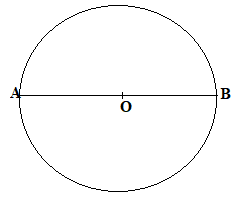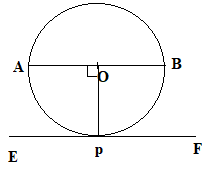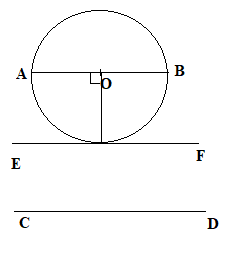QUESTION

# Draw a circle and two lines parallel to a given line that one is a tangent and the other is a secant to the circle.

Hint: In this question, first of all we will draw a circle and its diameter. We know that diameter is the largest scent. Therefore, considering diameter as the first line, we will draw a line outside the circle which is parallel to secant. After this, we will draw a tangent parallel to the diameter.

First of all we will draw the circle and its diameter.Here ‘O’ is the centre of the circle and AB is the diameter of the circle.
Next, we will draw a line CD parallel to AB.
To draw a line CD parallel to AB, we will make the alternate angle equal because we know that if two lines are parallel then the alternate angles are equal.After this we will make a tangent which is parallel to the diameter AB.
To make the tangent, we will first draw a perpendicular line from the diameter which intersects the circle at point P. Now, at this point we will make an angle of 90 degrees. On joining the two points, we get the tangent.So, the final diagram for the question is:$\because$ AB || CD and AB || EF.
So, we can say that:
AB || EF || CD.

Note: You have to solve this question step by step as we have solved here. Next thing is that you should know how to draw parallel lines. You should remember the different angles properties of when a line intersects two parallel lines. A tangent makes an angle of 90 degrees with the line joining the centre.